### THE INTEGRATION OF EXPONENTIAL FUNCTIONS

The following problems involve the integration of exponential functions. We will assume knowledge of the following well-known differentiation formulas :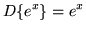,

where, and,

where a is any positive constant not equal to 1 andis the natural (base e) logarithm of a . These formulas lead immediately to the following indefinite integrals :

1.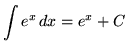2.As you do the following problems, remember these three general rules for integration :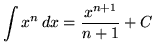,

where n is any constant not equal to -1,,

where k is any constant, and.

Because the integral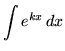,

where k is any nonzero constant, appears so often in the following set of problems, we will find a formula for it now using u-substitution so that we don't have to do this simple process each time. Begin by letting

u=kx

so that

du = k dx ,

or

(1/k)du = dx .

Now substitute into the original problem, replacing all forms of x, and getting.

We now have the following variation of formula 1.) :

3.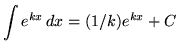.

The following often-forgotten, misused, and unpopular rules for exponents will also be helpful :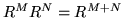and.

Most of the following problems are average. A few are challenging. Knowledge of the method of u-substitution will be required on many of the problems. Make precise use of the differential notation dx and du and always be careful when arithmetically and algebraically simplifying expressions.

• PROBLEM 1 : Integrate.

• PROBLEM 2 : Integrate.

• PROBLEM 3 : Integrate.

• PROBLEM 4 : Integrate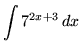.

• PROBLEM 5 : Integrate.

• PROBLEM 6 : Integrate.

• PROBLEM 7 : Integrate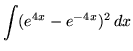.

• PROBLEM 8 : Integrate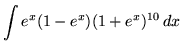.

• PROBLEM 9 : Integrate.

• PROBLEM 10 : Integrate.

• PROBLEM 11 : Integrate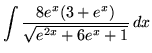.

• PROBLEM 12 : Integrate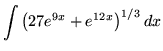.